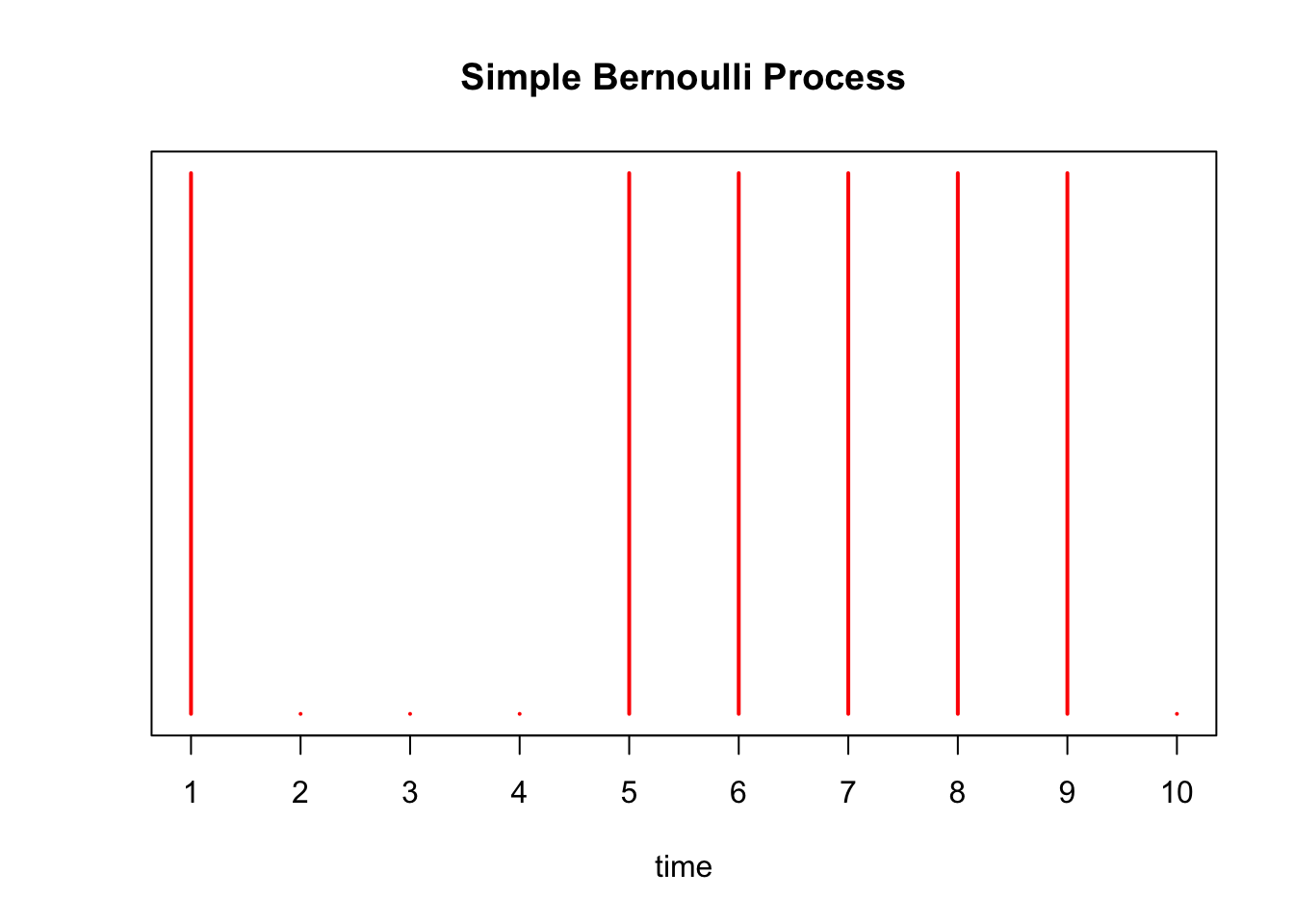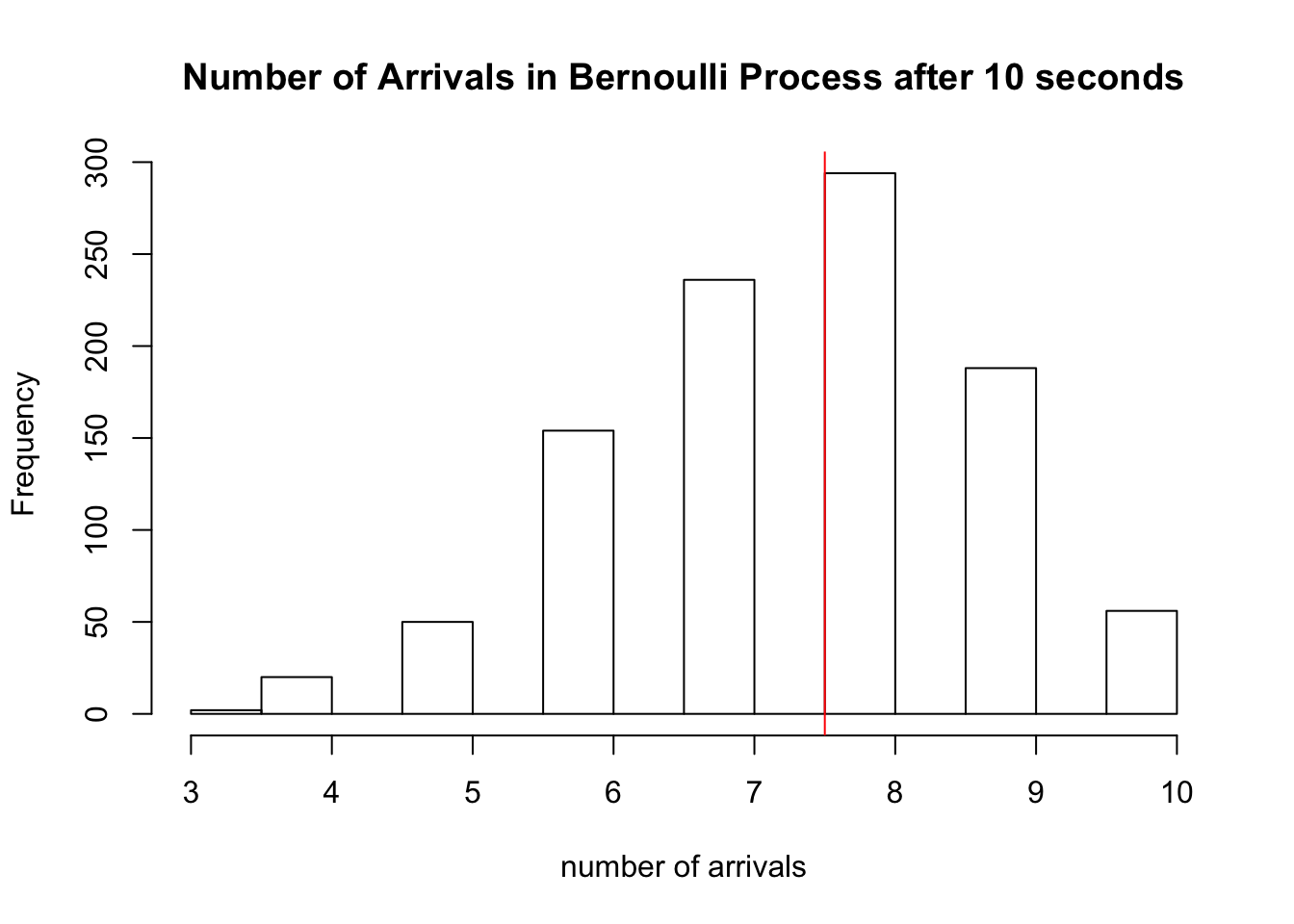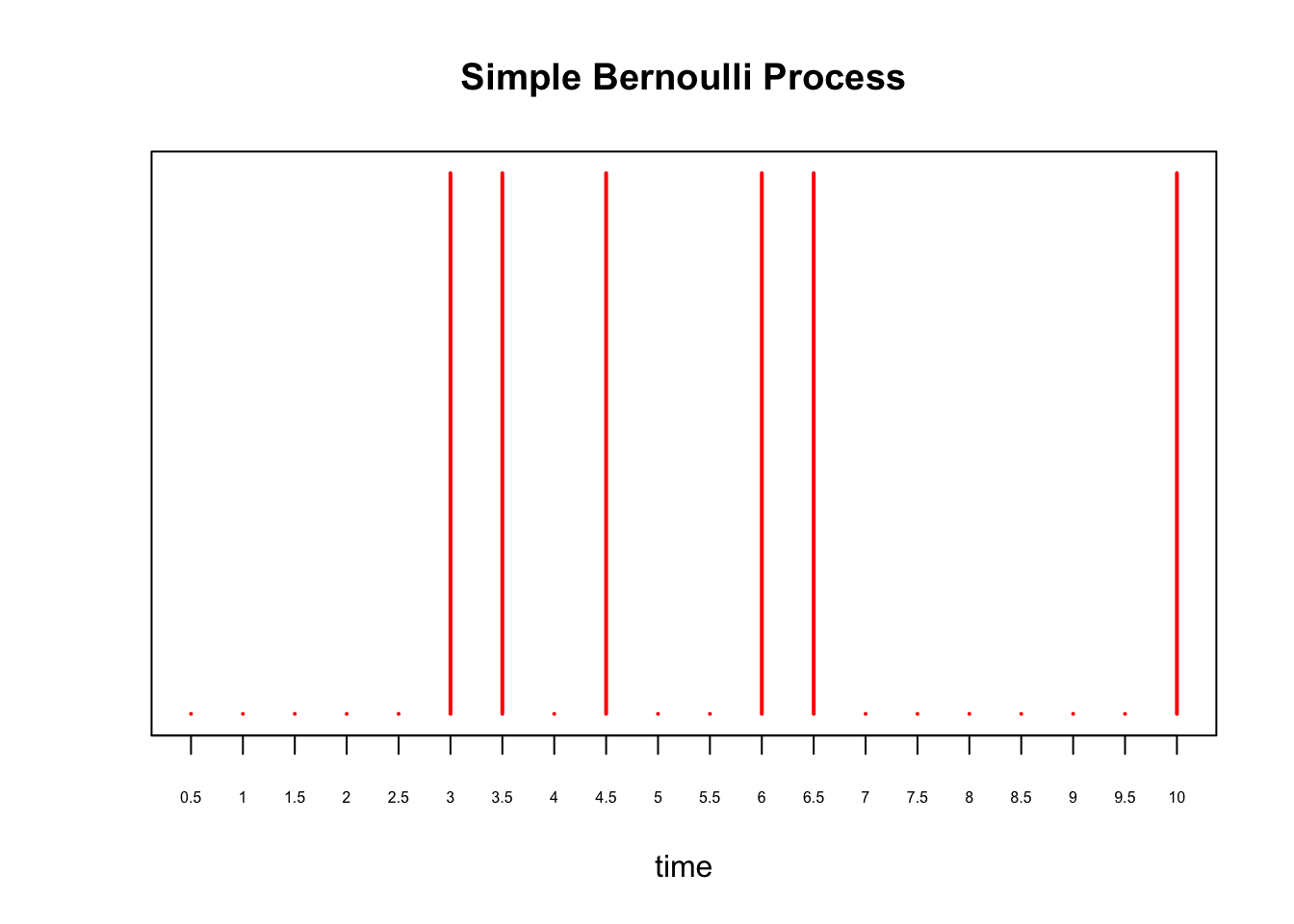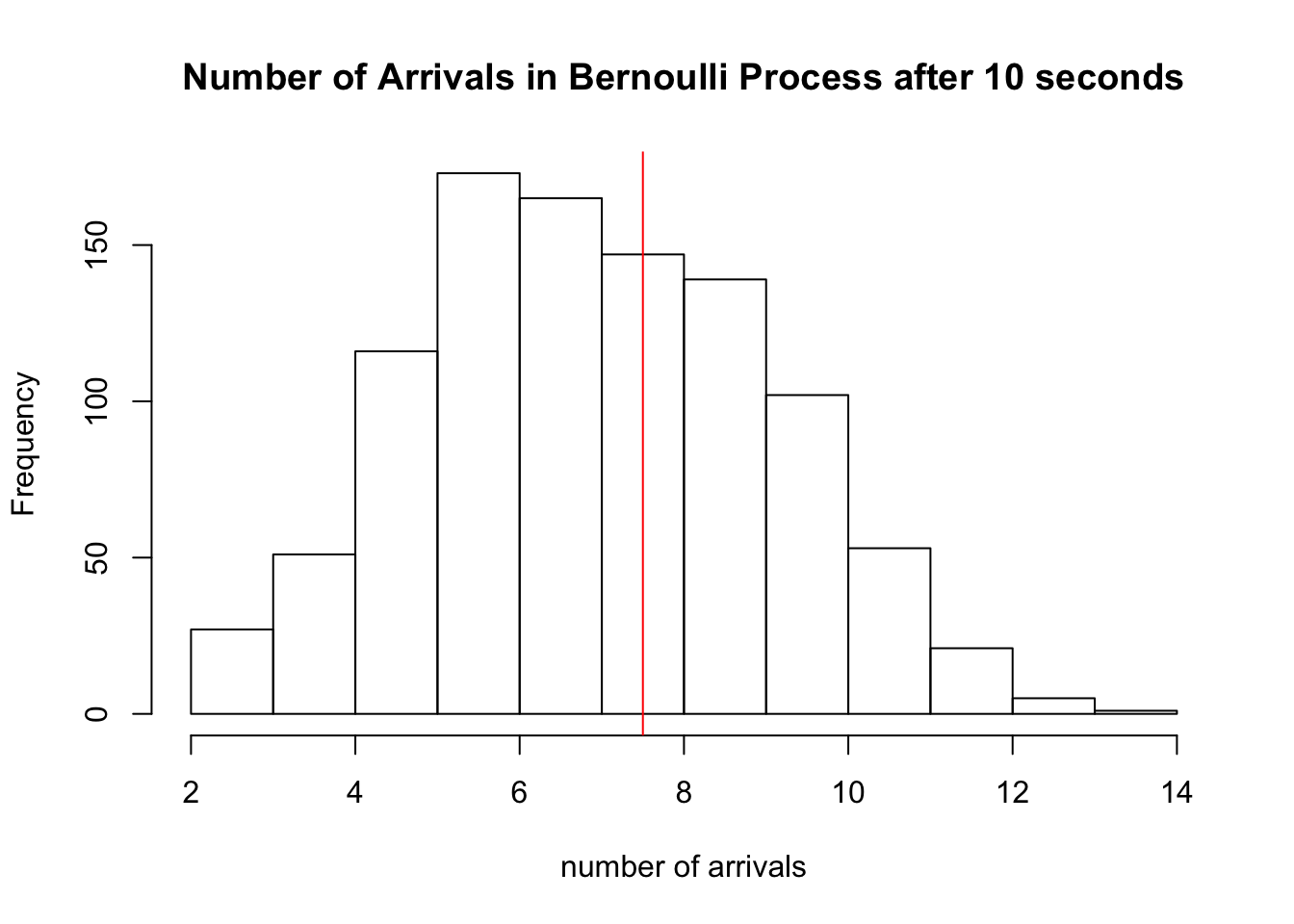Last updated: 2019-03-31

Checks: 5 1

Knit directory: fiveMinuteStats/analysis/

This reproducible R Markdown analysis was created with workflowr (version 1.2.0). The Report tab describes the reproducibility checks that were applied when the results were created. The Past versions tab lists the development history.

Great! Since the R Markdown file has been committed to the Git repository, you know the exact version of the code that produced these results.

Great job! The global environment was empty. Objects defined in the global environment can affect the analysis in your R Markdown file in unknown ways. For reproduciblity it’s best to always run the code in an empty environment.

The command set.seed(12345) was run prior to running the code in the R Markdown file. Setting a seed ensures that any results that rely on randomness, e.g. subsampling or permutations, are reproducible.

Great job! Recording the operating system, R version, and package versions is critical for reproducibility.

The following chunks had caches available:
• unnamed-chunk-5

To ensure reproducibility of the results, delete the cache directory bernoulli_poisson_process_cache and re-run the analysis. To have workflowr automatically delete the cache directory prior to building the file, set delete_cache = TRUE when running wflow_build() or wflow_publish().

Great! You are using Git for version control. Tracking code development and connecting the code version to the results is critical for reproducibility. The version displayed above was the version of the Git repository at the time these results were generated.

Note that you need to be careful to ensure that all relevant files for the analysis have been committed to Git prior to generating the results (you can use wflow_publish or wflow_git_commit). workflowr only checks the R Markdown file, but you know if there are other scripts or data files that it depends on. Below is the status of the Git repository when the results were generated:


Ignored files:
Ignored:    .Rhistory
Ignored:    .Rproj.user/
Ignored:    analysis/.Rhistory
Ignored:    analysis/bernoulli_poisson_process_cache/

Untracked files:
Untracked:  _workflowr.yml
Untracked:  analysis/CI.Rmd
Untracked:  analysis/gibbs_structure.Rmd
Untracked:  analysis/libs/
Untracked:  analysis/results.Rmd
Untracked:  analysis/shiny/tester/
Untracked:  docs/MH_intro_files/
Untracked:  docs/citations.bib
Untracked:  docs/figure/MH_intro.Rmd/
Untracked:  docs/hmm_files/
Untracked:  docs/libs/
Untracked:  docs/shiny/tester/



Note that any generated files, e.g. HTML, png, CSS, etc., are not included in this status report because it is ok for generated content to have uncommitted changes.

These are the previous versions of the R Markdown and HTML files. If you’ve configured a remote Git repository (see ?wflow_git_remote), click on the hyperlinks in the table below to view them.

File Version Author Date Message
html 34bcc51 John Blischak 2017-03-06 Build site.
Rmd 5fbc8b5 John Blischak 2017-03-06 Update workflowr project with wflow_update (version 0.4.0).
Rmd 391ba3c John Blischak 2017-03-06 Remove front and end matter of non-standard templates.
html fb0f6e3 stephens999 2017-03-03 Merge pull request #33 from mdavy86/f/review
Rmd d674141 Marcus Davy 2017-02-27 typos, refs
html c3b365a John Blischak 2017-01-02 Build site.
Rmd 67a8575 John Blischak 2017-01-02 Use external chunk to set knitr chunk options.
Rmd 5ec12c7 John Blischak 2017-01-02 Use session-info chunk.
Rmd f875d4f mbonakda 2016-02-06 section title
Rmd c2c298c mbonakda 2016-02-06 plot titles
Rmd 9ea41c3 mbonakda 2016-02-06 fix plot titles
Rmd 22b5540 mbonakda 2016-02-06 add bernoulli limit to poisson process

# Pre-requisites

This document assumes basic familiarity with the Poisson process.

# Overview

To gain intuition about the Poisson process, it is useful think of it as a continuous time version of the Bernoulli process. We first review some basics about the Poisson process and settle on notation before moving on to an illustrative example.

For a Poisson process, arrivals may occur at any arbitrary time $$t \geq 0$$. One way to specify a Poisson process is by the counting process $$N_t$$ denoting the number of arrivals up to and including time $$t$$. For a Poisson process with rate parameters $$\lambda$$, we have that $N_t \sim \text{Poisson}(\lambda t)$

Therefore, the expected number of time arrivals up to and including $$t$$ is equal to $$E[N_t] = \lambda t$$. In the examples that follow, we will assume our rate parameter $$\lambda$$ is $$0.75$$ arrivals per second, and we are interested in the number of arrivals after 10 seconds, $$N_{10}$$. In this example, we expect and average of $$10\times\lambda = 7.5$$ arrivals after $$10$$ seconds.

Now we will describe the discrete-time analog of the Poisson process, the Bernoulli process. Throughout the next section, our aim is to construct a Bernoulli process so that the expected number of arrivals after $$10$$ seconds matches the Poisson process above. We will then investigate what happens to the Bernoulli process as we increase the number of discrete events within the $$10$$ seconds while maintaining the expected number of arrivals at $$7.5$$.

# Bernoulli Process

The Bernoulli process is a sequence of independent random variables $$X_1,X_2,X_3,\ldots$$ where $$X_i$$ is $$1$$ with probability $$p$$ and $$0$$ with probability $$1-p$$. This is the mathematical abstraction of coin tossing.

Assume that we perform a Bernoulli trial every second for 10 seconds. We will think of each $$X_i=1$$ as an arrival. Recall that since our random variables $$X_i$$ are Bernoulli($$p$$), the sum $$S_{10} = \sum_{i=1}^{10} X_i$$ is Binomial(10, $$p$$). The expected value of $$S_{10}$$ is therefore $$10p$$ arrivals in $$10$$ seconds. To match the Poisson process above, we set $$p=0.75$$ so that $$E[S_{10}] = 7.5$$.

Below we simulate this process with $$p=0.75$$, drawing a red line at time point $$i$$ if $$X_i = 1$$:

X = rbinom(10, 1, 0.75)
plot(X, xaxt='n', yaxt='n', type='h', ylim=c(0,1),
col='red', lwd=2, ylab='', xlab='time', main='Simple Bernoulli Process')
axis(1, at = 1:10, labels=as.character(1:10))Version Author Date
34bcc51 John Blischak 2017-03-06
c3b365a John Blischak 2017-01-02

There are $$\sum_{i=1}^{10} X_i =$$ 6 arrivals in our experiment above. We simulate this Bernoulli process 1000 times below, and a plot a histogram of the $$S_{10}$$’s obtained from each trial. We draw a red line at the expected number of arrivals, 7.5.

hist(sapply(1:1000, function(x) sum(rbinom(10,1,0.75))), xlab='number of arrivals', main='Number of Arrivals in Bernoulli Process after 10 seconds')
abline(v=7.5, col='red')Version Author Date
34bcc51 John Blischak 2017-03-06
c3b365a John Blischak 2017-01-02

Note that for this process, time is discrete – events only happen at the integers $$1,2,\ldots 10$$, while the Poisson process models events happening at any time $$t \in [0,10]$$. We could next think of performing a Bernoulli trial more rapidly, like every 0.5 seconds, or every 0.01 seconds, and so on. Continuing in this fashion, we would eventually (in the limit) have a continuous time process where arrivals could happen at any moment in time. We will now investigate what happens as we “shrink” the Bernoulli process in this way, allowing for more trials within the $$10$$ seconds.

Instead of performing a Bernoulli trial at every second, assume we perform one at every $$\delta$$ seconds where, in this example, $$\delta < 1$$. This would make the total of trials $$n = \frac{10}{\delta}$$. Without making any other changes, $$S_{10}$$ would be a Binomial($$10 /\delta$$, $$0.75$$) random variable with the expected number of arrivals equal to: $E[S_{10}] = \frac{p\times 10}{\delta} = \frac{0.75\times 10}{\delta}$

To maintain the average of $$7.5$$ arrivals in $$10$$ second, we would need to shrink the probability so that \begin{align*} E[N_{10}] &= E[S_{10}] \\ 10 \lambda &= \frac{10\times p}{\delta} \\ \Rightarrow p &= \lambda \delta \\ &= 0.75\delta \end{align*}

where we substituted $$\lambda = 0.75$$ in the last line. Continuing our example, instead of every 1 second, supposed we perform a Bernoulli trial every $$\delta = 0.5$$ seconds for $$10$$ seconds. Then, to maintain the expected number of arrivals, we set $$p = 0.75\times 0.5 = 0.375$$. We plot this process below:

X = rbinom(20, 1, 0.375)
plot(X, xaxt='n', yaxt='n', type='h', ylim=c(0,1),
col='red', lwd=2, ylab='', xlab='time', main='Simple Bernoulli Process')
axis(1, at = 0:20, labels=as.character(seq(0, 10, by=0.5)), cex.axis=0.5)Version Author Date
34bcc51 John Blischak 2017-03-06
c3b365a John Blischak 2017-01-02

and show the histogram of 1000 simulations of $$S_{10}$$ for this process:

hist(sapply(1:1000, function(x) sum(rbinom(20,1,0.375))), xlab='number of arrivals', main='Number of Arrivals in Bernoulli Process after 10 seconds')
abline(v=7.5, col='red')Version Author Date
34bcc51 John Blischak 2017-03-06
c3b365a John Blischak 2017-01-02

# Discrete to Continuous

In the previous section we saw that as we perform more Bernoulli trials in the same $$10$$ seconds (i.e. a trial every $$\delta = 0.5$$ seconds), we had to decrease the success probability $$p$$ to maintain $$E[S_t] = E[N_t]$$, where $$N_t$$ was the number of arrivals from the Poisson process from the first section of this note.

The key result is this: as $$\delta \rightarrow 0$$, we have that the $$P(S_t = k) = P(N_t = k)$$ and, in this sense, the Bernoulli process converges to the Poisson process. We illustrate this in the plots below. Recall that $$N_t \sim \text{Poisson}(\lambda t)$$.

As we set $$\delta$$ to smaller and smaller values, in each plot we show a histogram of 5000 simulated $$S_{10}$$’s along with the Poisson density of $$N_{10}$$. We see that as $$\delta$$ gets smaller, the histogram converges to the Poisson density.

In the simulation above, we perform Bernoulli trials every $$\delta = 2^{-j}$$ seconds for increasing values of $$j$$. This characterizes how fast we “shrink” the Bernoulli process.

TOTAL.TIME   <- 10
lambda       <- 0.75 # poisson rate paramter
shrink.vec   <- 0:10
bern.process <- vector("list", length(shrink.vec))

for(idx in seq_along(shrink.vec)) {
j                   <- shrink.vec[idx]
p                   <- lambda*2^(-j) # bernoulli parameter
time                <- seq(0, TOTAL.TIME, by=2^(-j)) # time steps
bern.process[[idx]] <- sapply(1:5000, function(x) rbinom(length(time), 1, p))
}

# compare Bernoulli histogram to Poisson density
compare.density <- function(b.process, delta, inspect.at=10) {
num.row <- dim(b.process)

# compute bernoulli counts
tt      <- table(apply(b.process, 2, cumsum)[num.row,])

# compute poisson density
pois.density <- dpois(0:80, inspect.at*lambda)

# plot
plot(tt/sum(tt), main=paste("trial every", round(delta,4), "seconds"), xlab='number of arrivals after 10 seconds', ylab='density', xlim=c(0,15), ylim=range(c(tt/sum(tt), pois.density)))
lines(0:80,pois.density, 'b')
}

par(mfrow=c(2,2))
compare.density(bern.process[], 2^(-shrink.vec))
compare.density(bern.process[], 2^(-shrink.vec))
compare.density(bern.process[], 2^(-shrink.vec))
compare.density(bern.process[], 2^(-shrink.vec))Warning: The above code chunk cached its results, but it won’t be re-run if previous chunks it depends on are updated. If you need to use caching, it is highly recommended to also set knitr::opts_chunk\$set(autodep = TRUE) at the top of the file (in a chunk that is not cached). Alternatively, you can customize the option dependson for each individual chunk that is cached. Using either autodep or dependson will remove this warning. See the knitr cache options for more details.

sessionInfo()
R version 3.5.2 (2018-12-20)
Platform: x86_64-apple-darwin15.6.0 (64-bit)
Running under: macOS Mojave 10.14.1

Matrix products: default
BLAS: /Library/Frameworks/R.framework/Versions/3.5/Resources/lib/libRblas.0.dylib
LAPACK: /Library/Frameworks/R.framework/Versions/3.5/Resources/lib/libRlapack.dylib

locale:
 en_US.UTF-8/en_US.UTF-8/en_US.UTF-8/C/en_US.UTF-8/en_US.UTF-8

attached base packages:
 stats     graphics  grDevices utils     datasets  methods   base

loaded via a namespace (and not attached):
 workflowr_1.2.0 Rcpp_1.0.0      digest_0.6.18   rprojroot_1.3-2
 backports_1.1.3 git2r_0.24.0    magrittr_1.5    evaluate_0.12
 stringi_1.2.4   fs_1.2.6        whisker_0.3-2   rmarkdown_1.11
 tools_3.5.2     stringr_1.3.1   glue_1.3.0      xfun_0.4
 yaml_2.2.0      compiler_3.5.2  htmltools_0.3.6 knitr_1.21     

This site was created with R Markdown